What is Apparent Temperature? Description given in the National Digital Forecast Database (NDFD) Documentation: Apparent Temperature: The perceived temperature in degrees Fahrenheit derived from either a combination of temperature and wind (Wind Chill) or temperature and humidity (Heat Index) for the indicated hour. When the temperature at a particular grid point falls to 50 F or less, wind chill will be used for that point for the Apparent Temperature. When the temperature at a grid point rises above 80 F, the heat index will be used for Apparent Temperature. Between 51 and 80 F, the Apparent Temperature will be the ambient air temperature (Source). Definitions: Heat Index: High humidity combined with hot temperatures reduce the body's ability to cool itself increasing the risk of heat exhaustion, heat stroke, and other heat related health problems. The Heat Index, also referred to as apparent temperature, is an estimate of the temperature (in °F) that would similarly affect the body at normal humidity (about 20 percent). For example, if the actual temperature is 100°F with 40 percent relative humidity, the heat index is 110°F meaning the apparent temperature feels like 110°F to the body (Source). Equation: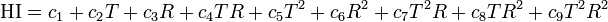where HI = Heat Index, T = Temperature (F), R = Relative Humidity (%), c1 = -42.38, c2 = 2.049, c3 = 10.14, c4 = -0.2248, c5 = -0.006838, c6 = -0.05482, c7 = 0.001228, c8 = 0.0008528, and c9 = -0.00000199 (Source). The source states this is accurate to within +/- 1.3 F. Wind Chill: The portion of the cooling of a human body caused by air motion. Air motion accelerates the rate of heat transfer from a human body to the surrounding atmosphere, especially when temperatures are below about 7°C (45°F) (Source). Equation:where Twc = Wind Chill Temperature, Ta = Atmospheric Temperature (F), and V = Air Speed (mph) (Source). The apparent temperature graph on this site uses essentially the same method used in the NDFD calculation, with one exception. I chose to restrict the usage of heat index a bit more by checking if the dew point temperature exceeds 12 C (54 F). If so, and if the air temperature exceeds 80 F, then the heat index is used as the apparent temperature. This is done because errors can start creeping into the results with high temps and low humidities, and with low humidity it technically violates the definition of heat index given above. An example is provided below, where all three conditions (heat index, wind chill, and air temperature) apply to give the apparent temperature: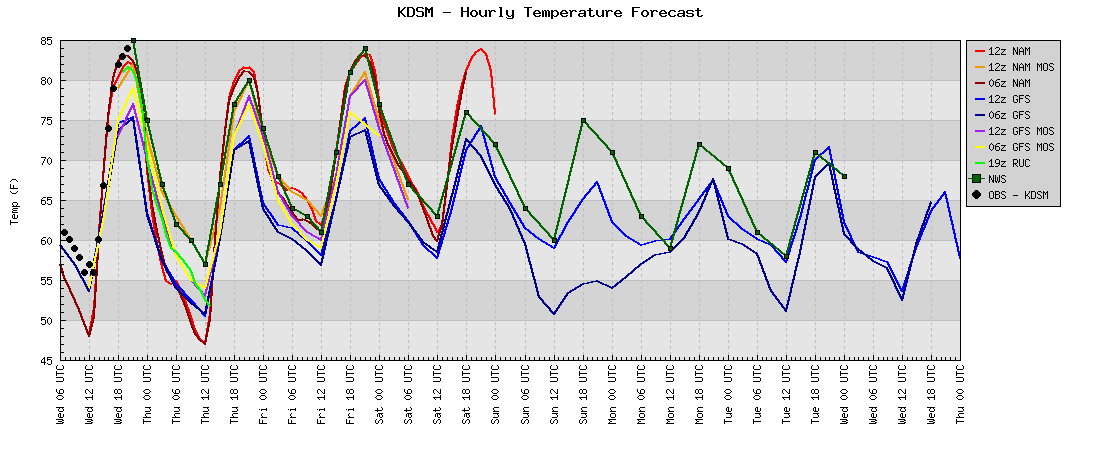Here, the temperature exceeds 80 F and dips below 50 F for a least a few hours during the time series...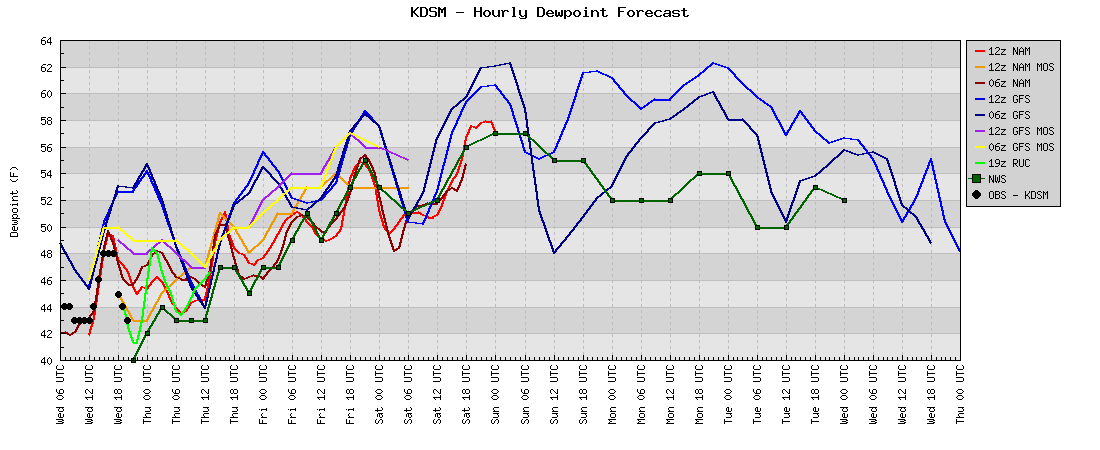While the dew point begins to exceed 54 F toward the end of the time series for some models when the air temperature exceeds 80 F...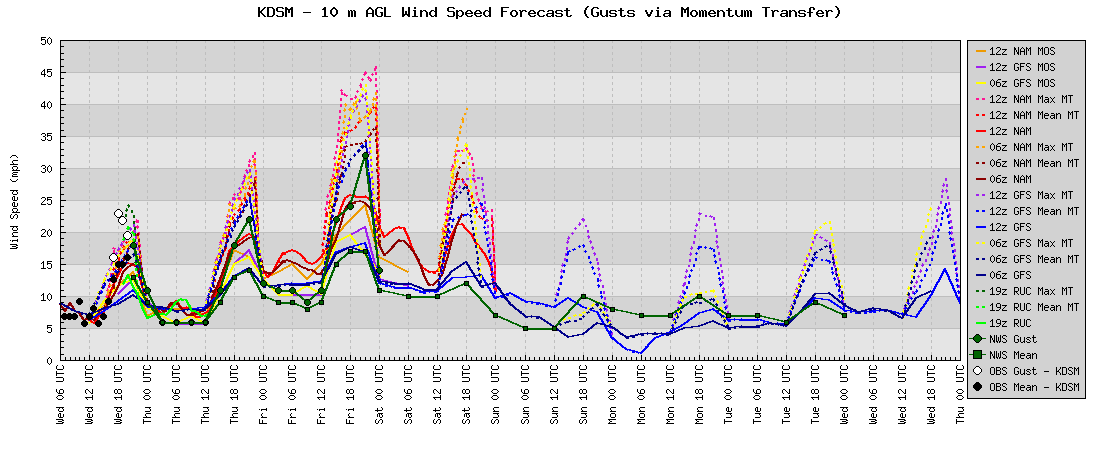Meanwhile, winds are greater than zero while the air temperature dips below 50 F...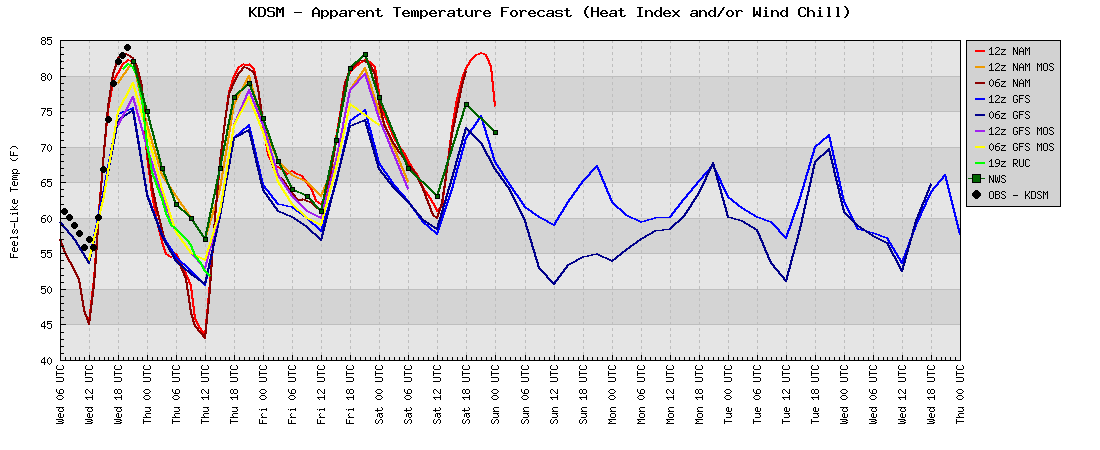The result is the apparent temperature plot. Hope some of you find this useful! Feedback is welcome!